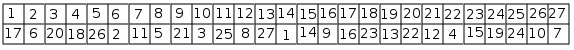# CAIA surprised me Part III The results

After finding symmetries, CAIA tries to find solutions to the magic cube problem, applying rules that enrich its formulation.

One of these rules states that, when there is a bijection between a set of unknowns and a set of values, the sum of the unknowns equals the sum of the values. Therefore, CAIA adds the constraint:

F(1)+F(2)+f(3)+…..+F(26)+F(27)=1+2+3+…..+26+27=378

When it substitutes the first three constraints in the preceding one, it sees that 3*VAL=378, so:

VAL=126

Using this value, and after many substitutions in the constraints, it finds several constraints with four unknowns, such as:

F(5)+F(23)=F(13)+F(15)

Later, it discovers a constraint with three unknowns:

F(5)+F(14)+F(23) = 42

Using the symmetries, it immediately adds two other constraints with three unknowns:

F(11)+F(14)+F(17) = 42

F(13)+F(14)+F(15) = 42

All the other symmetries lead to one of the three preceding constraints.

Finally, it happens that it has no more rule to apply. CAIA can choose between two possibilities:

1. It chooses an unknown, and it examines what happens when it substitutes it by one of its possible value. It carries on this task, instantiating an unknown, then generating new constraints, until it finds a contradiction or a solution. In both cases, it backtracks, instantiating the last unknown by the following possible value. CAIA is not very fast: it finds a solution every three seconds, taking into account that a new solution immediately leads to 47 other solutions, using the symmetries.

2. It writes a combinatorial program, cleverly using the constraints that it has found. This C program has 280 lines. CAIA compiles it, and executes it. Then, it finds 130 solutions every second.

In both cases, it chooses to begin backtracking with F(14), the small cube in the center of the large one. There are many solutions for each of the 27 possible values for F(14).

Here is one of these solutions:Its success is mainly due to its analysis of the problem formulation: it finds symmetries, and collects useful new constraints, particularly the three constraints with three unknowns. I did not know them myself, I had only found constraints with four unknowns. Naturally, when one knows that they exist, it is easy to prove them.

A human researcher, who would specifically solve this problem, could realize a more efficient system. However, the intelligence would be in his head, not in his system.

I wanted to compare CAIA with other problem solvers. Francis Sourd kindly gave this problem to two of the best general solvers for problems defined by constraints. ILOG Solver did not find a solution in a reasonable time, while ILOG CEPLEX found the first one after 14 hours. Incidentally, it shows that CEPLEX is excellent: the search space is enormous when it is impossible to manipulate the formulation of the problem. Therefore, it does not know the value of VAL, and all of its constraints have 10 unknowns.

CAIA solved this problem in a way that I did not know. As Pégoud with his plane, we must let our systems fend for themselves!

I doubt that, at the moment, there exists a general system that could approach the performances of CAIA for this problem, when it is placed in the same conditions: knowing only to find a bijection satisfying the 15 constraints given in part I. If I am wrong, I would be very interested in knowing more on this system.